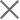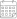Subscribe

### Indexes

December 2017 GDP Forecast | October GDP growth data is in: Georgia at 5.7%, Armenia surges to 17.6% year on year20 December 2017

ISET-PI updated its forecast of Georgia’s real GDP growth rate for the fourth quarter of 2017, and the first quarter of 2018. Here are the highlights of this month’s release:

Geostat updated its preliminary estimate of real GDP growth for the first and second quarters of 2017. The Q1 and Q2 GDP growth estimates were revised upward by 5.3% and 4.9%, respectively (0.2% higher than initial estimates). The third quarter estimates remained unchanged so far.

The real GDP growth rate reached 5.7 % y-o-y for October 2017. As a result, the estimated real GDP growth for the first ten months of 2017 was 4.9%.

ISET-PI’s real GDP growth forecast for the fourth quarter of 2017 remained at 4.6%.

Based on September’s data, we expect the annual growth in 2017 to be 4.8%.

ISET-PI forecasts GDP growth for the first quarter of 2018 to be 3.3%.